# Volume + area - math problems

#### Number of problems found: 359

• Height of the prism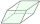The volume of the quadrilateral prism is 723.6 cm3. The base of this prism is a rhombus with a side 9 cm long and a corresponding height of 6.7 cm long. Find the height of the prism.
• Markus painterMarkus used ¾ liter of paint to cover 10 ½ square meters of wall. How many liters of paint is needed to cover 12 ¼ square meters of wall?
• GavinGavin had 3 gallons of orange juice ready to serve at the breakfast party. His family drank 2 ⅛ gallons. How much is left? Show your work with a numberline, area model or grid.
• The surfaceThe surface of the cylinder is 1570 cm2, its height is 15 cm. Find its volume and radius of the base.
• School modelThe beech school model of a regular quadrilateral pyramid has a base 20 cm long and 24 cm high. Calculate a) the surface of the pyramid in square decimeters, b) the mass of the pyramid in kilograms if the density of the beech is ρ = 0,8 g/cm ^ 3
• An experiment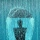The three friends agreed to the experiment. At the same time, they all took out an empty cylindrical container on the windowsill and placed it so that it was horizontal. Everyone lives in a different village, and each used a container with a different bot
• Right-angled triangle baseFind the volume and surface area of a triangular prism with a right-angled triangle base if the length of the prism base legs are 7.2 cm and 4.7 cm and the height of a prism is 24 cm.
• Frustrum - volume, areaCalculate the surface and volume of the truncated cone, the radius of the smaller figure is 4 cm, the height of the cone is 4 cm and the side of the truncated cone is 5 cm.
• Frustrum - volume, area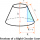Calculate the surface and volume of a truncated rotating cone with base radii of 8 cm and 4 cm, height 5 cm.
• Regular square prismThe volume of a regular square prism is 192 cm3. The size of its base edge and the body height is 1: 3. Calculate the surface of the prism.
• Trapezoidal base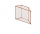Calculate the surface and volume of a quadrilateral prism with a trapezoidal base, where a = 7 cm, b = 4 cm, c = 5 cm, d = 4 cm, height of trapezium v = 3.7 cm and the height of the prism h = 5 cm.
• Axial sectionCalculate the volume and surface of a cone whose axial section is an equilateral triangle with side length a = 18cm.
• Truncated pyramid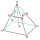The truncated regular quadrilateral pyramid has a volume of 74 cm3, a height v = 6 cm, and an area of the lower base 15 cm2 greater than the upper base's content. Calculate the area of the upper base.
• The tetrahedron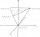Calculate the surface area and volume of a regular tetrahedron 4.9 cm high, the base edge has a length of 6 cm.
• The blockThe block, the edges formed by three consecutive GP members, has a surface area of 112 cm2. The sum of the edges that pass through one vertex is 14 cm. Calculate the volume of this block.
• Base diagonalIn a regular 4-sided pyramid, the side edge forms an angle of 55° with the base's diagonal. The length of the side edge is eight meters. Calculate the surface area and volume of the pyramid.
• Side edgesThe regular 4-sided pyramid has a body height of 2 dm, and the opposite side edges form an angle of 70°. Calculate the surface area and volume of the pyramid.
• Surface and volume - cubeFind the surface and volume of a cube whose wall diagonal is 5 cm long.
• Triangular prismThe regular triangular prism has a base edge of 8.6 dm and a height of 1.5 m. Finf its volume and surface area.
• The volumeThe volume of the cone is 94.2dm³, the radius of the base is 6 dm Calculate the surface of the cone.

Do you have an exciting math question or word problem that you can't solve? Ask a question or post a math problem, and we can try to solve it.

We will send a solution to your e-mail address. Solved examples are also published here. Please enter the e-mail correctly and check whether you don't have a full mailbox.

Tip: Our volume units converter will help you with the conversion of volume units. Volume - math problems. Area - math problems.